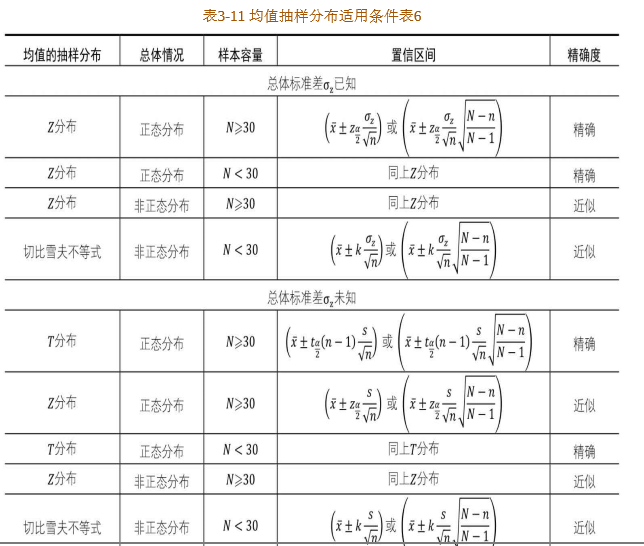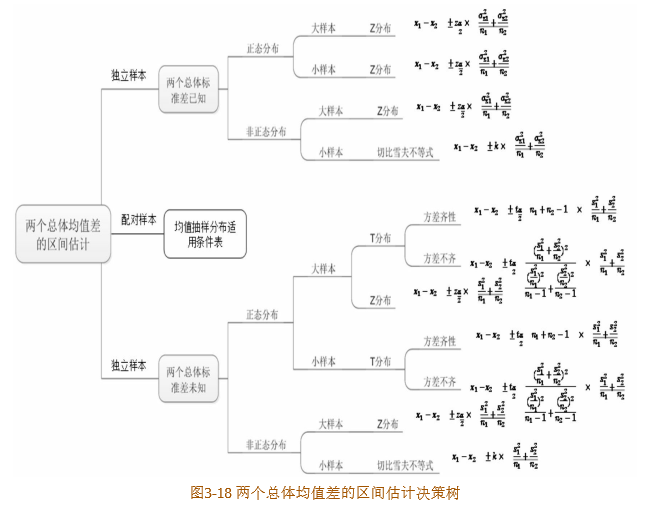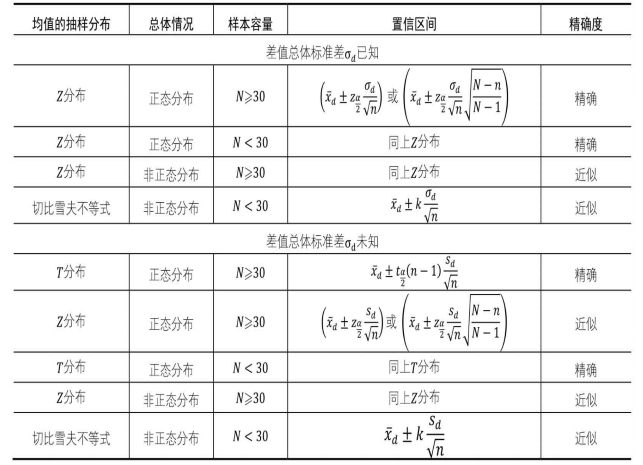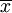• ## 参数估计（点估计和区间估计）

万次阅读 多人点赞 2019-09-06 12:07:06
1.点估计就是用样本统计量来估计总体参数。 概念理解：当我们想知道某一总体的某个指标的情况时，测量整体该指标的数值 的工作量太大，或者不符合实际，这时我们可以采用抽样的方法选取一部分样本测量出他们数值，...
“参数估计是以抽样分布为中介，用样本的参数特征对总体的参数进行数值估计的过程。”
一、点估计 1.点估计就是用样本统计量来估计总体参数。 概念理解：当我们想知道某一总体的某个指标的情况时，测量整体该指标的数值 的工作量太大，或者不符合实际，这时我们可以采用抽样的方法选取一部分样本测量出他们数值，然后用样本统计量的值来估计总体的情况。 例如：想了解一个学校学生的身高情况，就可以随机抽取一部分学生测量他们的身高，得到一个平均值，再用这个样本的均值去估计整体学生的身高情况，就是点估计。
常用的点估计有：用样本均值估计总体均值，用样本方差估计总体方差，用样本的分位数估计总体分位数，用样本的中位数估计总体的中位数。
2.点估计方法 矩估计法、顺序统计量法、最大似然法、 最小二乘法（对于点估计方法，放在另一篇文章中详细介绍）
3.由于用样本推断总体的过程一定存在估计误差，而点估计的估计误差无法衡量，所以点估计主要用于为定性研究提供数据参考，或者在对于总体参数估计精度要求不高时使用。
二、区间估计 1.区间估计就是在点估计的基础上，给出总体参数估计的一个区间范围，该区间通常由样本统计量加减估计误差得到。 另外一种说法，区间估计是从点估计值和抽样标准误差出发，按给定的概率值建立包含待估参数的区间，这个给定的概率值称为置信度或置信水平，这个建立起来的包含待估计参数的区间称为置信区间。
2.关于置信水平（置信度）、置信区间和显著性水平： 置信区间是根据样本信息推导出来的可能包含总体参数的数值区间，置信水平表示置信区间的可信度；例如某学校学生的平均身高的区间估计：有95%的置信水平可以认为该校学生的平均身高为1.4米到1.5米之间，（1.4,1.5）为置信区间，95%是置信水平，即有95%的信心认为这个区间包含该校学生的平均身高。 置信水平用百分数表示，表示成（1-a）100%；a指的是显著性水平，表示总体参数不落在置信区间的可能性。
3.关于置信区间的计算： 通过部分样本来计算总体参数的一个置信区间有以下步骤： a.明确要解决的问题，要估计的指标或参数是什么， b.求抽样样本的平均值和标准误差， 注意区分标准差和标准误差:标准差反映的是整个样本对样本平均数的离散程度，标准差等于方差开根号；标准误差反映的是样本平均数对总体平均数的变异程度，标准误差等于样本标准差除n的开根号。 c.确定需要的置信水平， d.查询z表，得到z值， e. 计算置信区间，[a,b],a=样本均值-z标准误差，b=样本均值+z标准误差。
区间估计分为一个总体参数的估计和两个总体参数的估计
4.一个总体参数的区间估计：总体均值的区间估计，总体方差的区间估计，总体比例的区间估计；
4.1总体均值的区间估计： 均值抽样分布即样本均值组成的抽样分布，总体参数的估计方法跟样本均值的抽样分布有关； Z分布其实就是标准正态分布，如果样本均值组成的抽样分布服从正态分布，那么将该正态分布标准化后即可得到Z分布， Z分布的适用条件有两种：一是总体服从正态分布且总体标准差已知；二是总体分布未知，但是样本容量大于或等于30； T分布：对于服从正态分布的总体且总体标准差未知的情况下 ，T分布是非常适用的均值抽样分布类型； 切比雪夫不等式：对于非正态分布总体或总体分布未知并且小样本的情况下，只能用切比雪夫不等式来近似估计总体均值的置信区间。截图来自《人人都会数据分析：从生活实例学统计》
4.2 总体方差的区间估计： 总体方差的区间估计要用到卡方分布，如果数据总体服从正态分布，从中抽取样本容量为n的样本，样本方差为s^2,那么包含样本方差的卡方统计量服从自由度为n-1的卡方分布。卡方统计量是由总体方差和样本方差的比值组成的统计量，用于总体方差的区间估计。 卡方统计量的计算公式：

χ

α

2

(

n

−

1

)

=

(

n

−

1

)

s

2

σ

z

2

\chi^2_\alpha(n-1)=\frac{(n-1)s ^2}{\sigma ^2_z}

总体方差的双侧置信区间估计公式为：

(

n

−

1

)

s

2

χ

α

2

2

(

n

−

1

)

≤

σ

z

2

≤

(

n

−

1

)

s

2

χ

1

2

−

α

2

(

n

−

1

)

\frac{(n-1)s^2}{\chi ^2_\frac{\alpha}{2}(n-1)} \leq \sigma ^2_z \leq \frac{(n-1)s ^2}{\chi ^2_1-\frac{\alpha}{2} (n-1)}

其中带有a/2的为下标； 如果是单侧置信区间的话，只需要取上面式子的前半部分或者后半部分，并将a/2改成a即可得到单侧置信区间。
4.3 总体比例的区间估计： 或者叫总体比率的区间估计，跟二项分布有关，二项分布的理论是：事件发生概率是p,进行n次实验，其中x次实验该事件发生，则发生次数的概率分布服从二项分布；均值、方差为np,npq。 若将发生的次数转换成比率（x/n），则比率的概率分布也服从二项分布。 二项分布的特性：当抽取的样本容量n很大，是大 样本，使得np和nq（q为事件不发生的概率，等于1-p）的值都大于 5， 此时二项分布将近似于正态分布。 由于事件发生比率x/n服从二项分布，所以如果比率的二项分布近似于正态分布，就可以得到不利的区间估计。
在事件发生概率p已知的情况下，总体比率

p

z

ˉ

\bar{p_z}

在置信度为1-a时，总体比率的置信区间为：

p

y

ˉ

±

Z

α

2

p

(

1

−

p

)

n

\bar{p_y} \pm Z_\frac{\alpha}{2} \sqrt{\frac{p(1-p)}{n}}

其中，

p

y

ˉ

\bar{p_y}

为样本比率，

p

z

ˉ

\bar{p_z}

为总体比率， 当事件发生概率p未知，可用样本中事件发生的概率即样本比率代替。
5. 两个总体参数的区间估计： 两个总体均值之差的估计，两个总体方差比的区间估计 两个总体与多个总体参数的区间估计在实际生活中的应用不是很多，更常用的是两个总体和多个总体参数的假设检验。 区间估计虽不常用，但是其与假设检验的应用原理是想通的。
5.1 两个总体均值之差的区间估计： 可以将单个总体均值的抽样分布推广到两个总体均值差的抽样分布，然后利用两个总体均值差的抽样分布推导出两个总体均值差的置信区间公式。 方差齐性/方差不齐：对于配对样本来说其方差可被认为是想等的，即方差齐性。截图来自《人人都会数据分析：从生活实例学统计》
独立样本和配对样本： 独立样本：是指如果从一个总体中选取样本，抽样形式无论怎样改变都不会影响从另一个总体中抽取样本的概率，则这两个随机样本为独立样本； 配对样本：是指如果从一个总体中抽取样本的行为以某种方式决定了从另一个总体中抽取样本的概率，则这两个样本为成对样本或配对样本。
均值和方差的特点： 两个总体合并（相加或相减），那么合并后的总体均值等于原来两个总体的均值之和或均值之差；而合并后的总体方差都等于两个总体方差之和。
差值抽样分布可以看做单个总体的均值抽样分布，因此可套用“均值抽样分布适用条件表”，将公式修改一下即可：截图来自《人人都会数据分析：从生活实例学统计》
5.2 两个总体方差比的区间估计 F分布可用于求取两个正态分布总体方差比的置信区间。 F统计量可被看做是两个卡方统计量的商，F分布也被称为方差比分布。因为卡方分布要求总体服从正态分布，所以F分布也要求F统计量的两个总体都服从正态分布。 当给定置信水平时，可推出两个正态分布总体方差比的置信区间。
三、样本量的确定
1总体均值区间估计的样本量确定 在总体标准差已知的情况下，如果数据总体服从正态分布，则样本均值的抽样分布适用Z分布，就可以利用总体均值的置信区间公式来计算样本容量，总体均值的置信区间为：

x

ˉ

±

Z

α

2

σ

n

\bar{x}\pm Z_\frac{\alpha}{2} \frac{\sigma}{\sqrt{n}}

或

x

ˉ

±

Z

α

2

σ

n

N

−

n

N

−

1

\bar{x}\pm Z_\frac{\alpha}{2} \frac{\sigma}{\sqrt{n}} \sqrt{\frac{N-n}{N-1}}

则总体均值的区间估计误差为：

Δ

μ

=

Z

α

2

σ

n

\Delta\mu=Z_\frac{\alpha}{2} \frac{\sigma}{\sqrt{n}}

进而可以求得样本容量的公式：

n

=

(

Z

α

2

σ

Δ

μ

)

2

n=(\frac{Z_\frac{\alpha}{2} \sigma}{\Delta\mu})^2

以上是总体标准差已知时，当总体标准差未知时，一是可以用样本标准差代替，但是前提条件是样本容量要大于等于30；二是可以用过去试点调查的样本标准差代替；三是，如果知道总体数据中的最大和最小值，可用四分之一的最大与最小值的差值来代替总体标准差。
2.总体方差区间估计的样本量确定 总体方差的区间估计适用的抽样分布为卡方分布。卡方统计量为:

χ

2

=

(

n

−

1

)

s

2

σ

2

\chi^2=\frac{(n-1)s ^2}{\sigma ^2}

由卡方分布的性质可知，当样本量足够大时，卡方分布近似于正态分布。卡方分布的均值为自由度（n-1）,卡方分布的方差为两倍的自由度2（n-1），那么在大样本的情况下，总体方差的置信区间为：

s

2

=

±

Z

α

2

s

2

2

n

s^2=\pm Z_\frac{\alpha}{2} s^2 \sqrt{\frac{2}{n}}

则总体方差的估计精度为：

Δ

σ

2

=

Z

α

2

s

2

2

n

\Delta \sigma^2=Z_\frac{\alpha}{2} s^2 \sqrt{\frac{2}{n}}

由此可得到样本容量公式为：

n

=

2

Z

α

2

s

2

Δ

σ

2

n=\frac{\sqrt{2} Z_\frac{\alpha}{2} s^2}{\Delta \sigma^2}

3.总体比率区间估计的样本量确定 在确定总体比率的区间估计时，利用的是二项分布近似于正态分布的性质，即当抽取的样本量n很大时,是大样本，使得np>5且nq>5(p是事件发生的概率，q是事件不发生的概率，q=1-p)时，二项分布近似于正态分布。 总体比率的置信区间为：

p

y

ˉ

±

Z

α

2

p

(

1

−

p

)

n

\bar{p_y} \pm Z_\frac{\alpha}{2} \sqrt{\frac{p(1-p)}{n}}

则总体比率的估计误差为：

Δ

p

z

ˉ

=

Z

α

2

p

(

1

−

p

)

n

\Delta \bar{p_z} =Z_\frac{\alpha}{2} \sqrt{\frac{p(1-p)}{n}}

由此可得到样本容量为：

n

=

Z

α

2

2

p

(

1

−

p

)

Δ

p

z

ˉ

2

n=\frac{Z_\frac{\alpha}{2} ^2 p(1-p)}{\Delta \bar{p_z} ^2}

注：本文主要参考《人人都会数据分析：从生活实例学统计》
展开全文区间估计
• 根据参数估计的性质不同，可以分成两种类型：点估计区间估计。 点估计 点估计就是用样本统计量的某一具体数值直接推断未知的总体参数。例如，在进行有关小学生身高的研究中，随机抽取1000名小学生并计算出他们的...
参数估计就是根据样本统计量的数值对总体参数进行估计的过程。根据参数估计的性质不同，可以分成两种类型：点估计和区间估计。
点估计
点估计就是用样本统计量的某一具体数值直接推断未知的总体参数。例如，在进行有关小学生身高的研究中，随机抽取1000名小学生并计算出他们的平均身高为1.46米。如果直接用这个1.46米代表所有小学生的平均身高，那么这种估计方法就是点估计。 对总体参数进行点估计常用的方法有两种：矩估计与最大似然估计，其中最大似然估计就是我们实际中使用非常广泛的一种方法。 按这两种方法对总体参数进行点估计，能够得到相对准确的结果。如用样本均值X估计总体均值，或者用样本标准差S估计总体标准差σ。 点估计有一个不足之处，即这种估计方法不能提供估计参数的估计误差大小。对于一个总体来说，它的总体参数是一个常数值，而它的样本统计量却是随机变量。当用随机变量去估计常数值时，误差是不可避免的，只用一个样本数值去估计总体参数是要冒很大风险的。因为这种误差风险的存在，并且风险的大小还未知，所以，点估计主要为许多定性研究提供一定的参考数据，或在对总体参数要求不精确时使用，而在需要用精确总体参数的数据进行决策时则很少使用。
区间估计
区间估计就是在推断总体参数时，还要根据统计量的抽样分布特征，估计出总体参数的一个区间，而不是一个数值，并同时给出总体参数落在这一区间的可能性大小，概率的保证。还是举小学生身高的例子，如果用区间估计的方法推断小学生身高，则会给出以下的表达：根据样本数据，估计小学生的平均身高在1.4~1.5米之间，置信程度为95%，这种估计就属于区间估计。
展开全文统计学
• ## 参数估计

千次阅读 2019-09-02 22:09:01
参数估计包括点估计区间估计两类。 点估计 点估计是以抽样得到的样本指标作为总体指标的估计量，并以样本指标的实际值直接作为总体未知参数的估计值的一种推断方法。 点估计(point estimate)是用样本统计量的某个...
参数估计包括点估计和区间估计两类。
点估计
点估计是以抽样得到的样本指标作为总体指标的估计量，并以样本指标的实际值直接作为总体未知参数的估计值的一种推断方法。
点估计(point estimate)是用样本统计量的某个取值直接作为总体参数的估计值。例如，用样本均值x直接作为总体均值μ的估计值，用样本方差s2直接作为总体方差σ2的估计值。点估计的方法有：矩估计法、顺序统计量法、最大似然法、最小二乘法。
矩估计法：矩是指以期望为基础而定义的数字特征，一般分为原点矩和中心矩。设X为随机变量，对任意正整数k，称E(Xk)为随机变量X的k阶原点矩，记为：

m

k

=

E

(

X

k

)

m_k=E(X^k)

当k=1时，m1=E(X)=μ，可见一阶原点矩为随机变量X的数学期望。
把Ck=E[X-E(X)]k称为以E(X)为中心的k阶中心矩。显然，当k=2时，C2=E[X-E(x)]2=σ2，可见二阶中心矩为随机变量X的方差。
顺序统计量法：用样本中位数估计总体的数学期望的方法称数学期望的顺序统计量估计法。顺序统计量估计法的优点是计算简便，且中位数不易受个别异常数据的影响．如果一组样本值某一数据异常(如过于小或过于大)，则这个异常数据可能是总体的随机性造成的，也可能是受外来干扰造成的(如工作人员粗心，记录错误)，当原因属于后者，用样本平均值估计E(x)显然受到影响，但用样本中位数估计总体期望时，由于一个(甚至几个)异常的数据不易改变中位数的取值，所以估计值不易受到影响。
最大似然法(Maximum Likelihood)：它用来求一个样本集的相关概率密度函数的参数。
最小二乘法(generalized least squares)：是一种数学优化技术，它通过最小化误差的平方和找到一组数据的最佳函数匹配。 最小二乘法是用最简的方法求得一些绝对不可知的真值，而令误差平方之和为最小。 最小二乘法通常用于曲线拟合。很多其他的优化问题也可通过最小化能量或最大化熵用最小二乘形式表达。
区间估计
区间估计(interval estimate)是在点估计的基础上，给出总体参数估计的一个区间范围，该区间通常由样本统计量加减估计误差得到。与点估计不同，进行区间估计时，根据样本统计量的抽样分布可以对样本统计量与总体参数的接近程度给出一个概率度量。
区间估计中，由样本统计量所构造的总体参数的估计区间称为置信区间(confidence interval)，其中区间的最小值称为置信下限，最大值称为置信上限。
如果将构造置信区间的步骤重复多次，置信区间中包含总体参数真值的次数所占的比例称为置信水平(confidence level)，也称为置信度或置信系数(confidence coefficient)。
区间估计的正确理解方式：区间估计并不是总体参数落在某个区间的概率，而是抽取的多个样本中有多大的概率包含总体参数，由此通过概率可以知道在多次抽样得到的区间中大概有多少个区间包含了参数的真值。
一个总体参数的区间估计
研究一个总体时，所关心的参数主要有总体均值μ、总体比例π和总体方差σ2等。
总体均值的区间估计 对总体均值进行区间估计时，需要考虑总体是否为正态分布，总体方差是否已知，用于构造估计量的样本是大样本（通常要求n≥30）还是小样本（n<30）等几种情况。下面分两种情况来分析： （1）正态总体、方差已知，或非正态总体、大样本 当总体服从正态分布且方差已知，或总体非正态分布但样本为大样本时，样本均值x的抽样分布服从正态分布，其数学期望为总体均值μ，方差为σ2/n。样本均值经过标准化后的随机变量则服从正态分布，即

z

=

x

‾

−

μ

σ

/

n

∼

N

(

0

,

1

)

z=\frac{\overline{x}-\mu}{\sigma/\sqrt{n}}\thicksim N(0,1)

根据式上式和正态分布的性质可以得出总体均值μ在1-α置信水平下的置信区间为：

x

‾

±

z

α

/

2

σ

n

\overline{x}\pm{z_{\alpha/2}}\frac{\sigma}{\sqrt{n}}

（2）正态总体、方差未知、小样本 在总体服从正态分布的情况下，如果总体方差σ2未知，且样本较小的情况下，需要用样本方差s2代替σ2。这时，样本均值经过标准化以后的随机变量服从自由度为(n-1)的t分布，即

t

=

x

‾

−

μ

s

/

n

∼

t

(

n

−

1

)

t=\frac{\overline{x}-\mu}{s/\sqrt{n}}\thicksim{t(n-1)}

因此需要采用t分布来建立总体均值μ的置信区间。根据t分布建立的总体均值μ在1-α置信水平下的置信区间为：

x

‾

±

t

α

/

2

s

n

\overline{x}\pm{t_{α/2}\frac{s}{\sqrt{n}}}

总体比例的区间估计 在大样本的前提下，样本比例p的抽样分布可用正态分布近似。p的数学期望为E§=π，p的方差为σ2p=π(1-π)/n。而样本比例经标准化后的随机变量则服从标准正态分布，即

z

=

p

−

π

π

(

1

−

π

)

/

n

∼

N

(

0

,

1

)

z=\frac{p-\pi}{\sqrt{\pi(1-\pi)/n}}\thicksim{N(0,1)}

与总体均值的区间估计类似，在样本比例p的基础上加减估计误差zα/2σp，即得总体比例π在1-α置信水平下的置信区间为：

p

±

z

α

/

2

π

(

1

−

π

)

n

p\pm{z_{\alpha/2}\sqrt{\frac{\pi(1-\pi)}{n}}}

当通过上式计算总体比例π的置信区间时，π值应该是已知的。但实际情况不然，π值恰好是要估计的，所以需要用样本比例p来代替π。这种情况下，总体比例的置信区间可表示为：

p

±

z

α

/

2

p

(

1

−

p

)

n

p\pm{z_{\alpha/2}\sqrt{\frac{p(1-p)}{n}}}

总体方差的区间估计 对于总体方差的估计，这里只讨论正态总体方差的估计。根据样本方差的抽样分布可知，样本方差服从自由度为n-1的χ2分布。因此用χ2分布构造总体方差的置信区间。 总体方差σ2在1-α置信水平下的置信区间为：

(

n

−

1

)

s

2

χ

α

/

2

2

≤

σ

2

≤

(

n

−

1

)

s

2

χ

1

−

α

/

2

2

\frac{(n-1)s^2}{{\chi^2_{\alpha/2}}}\leq\sigma^2\leq\frac{(n-1)s^2}{\chi^2_{1-\alpha/2}}

两个总体参数的区间估计后续讨论。
样本量的确定
通过区间估计可以了解到样本量的选择对于问题的求解至关重要，大样本（n≥30）和小样本（n<30）求解的方法不同。同样是大样本选择多大的样本来估计参数比较合适？
通常，样本量的确定与可以容忍的置信区间的宽度以及对此区间设置的置信水平有一定关系。因此如何确定一个适当的样本量，也是抽样估计中需要考虑的问题。
估计总体均值时样本量的确定
总体均值的置信区间是由样本均值x和估计误差两部分组成的。在重复抽样或无限总体抽样条件下，估计误差为：

z

α

/

2

σ

n

z_{\alpha/2}\frac{\sigma}{\sqrt{n}}

其中zα/2的值和样本n共同确定了估计误差的大小。当确定了置信水平1-α，zα/2的值就确定了。对于给定的zα/2的值和总体标准差σ，就可以确定任一希望的估计误差所需要的样本量。令E代表所希望达到的估计误差，即：

E

=

z

α

/

2

σ

n

E=z_{\alpha/2}\frac{\sigma}{\sqrt{n}}

通过上式可以推导出确定样本量的公式如下：

n

=

(

z

α

/

2

)

2

σ

2

E

2

n=\frac{(z_{\alpha/2})^2\sigma^2}{E^2}

式中的E值是使用者在给定的置信水平下可以接受的估计误差，zα/2的值可直接由区间估计中所用到的置信水平确定。当σ未知时，可以用样本的标准差来代替；也可以用试验调查的办法，选择一个初始样本，以该样本的标准差作为σ的估计值。
从上式可以看出，样本量与置信水平成正比，在其他条件不变的情况下，置信水平越大，所需要的样本量也就越大；样本量与总体方差成正比，总体的差异越大，所要求的样本量也越大；样本量与估计误差的平方成反比，即可以接受的估计误差的平方越大，所需的样本量就越小。
估计总体比例时样本量的确定
与估计总体均值时样本量确定的方法类似，在重复抽样或无限总体抽样条件下，估计总体比例置信区间的估计误差为：

z

α

/

2

π

(

1

−

π

)

n

{z_{\alpha/2}\sqrt{\frac{\pi(1-\pi)}{n}}}

由上式可知，zα/2的值、总体比例π和样本量n共同确定了估计误差的大小。令E代表所希望达到的估计误差，即：

E

=

z

α

/

2

π

(

1

−

π

)

n

E=z_{\alpha/2}\sqrt{\frac{\pi(1-\pi)}{n}}

据此可以推导出重复抽样或无限总体抽样条件下确定样本量的公式如下：

n

=

(

z

α

/

2

)

2

π

(

1

−

π

)

E

2

n=\frac{(z_{\alpha/2})^2\pi(1-\pi)}{E^2}

式中的估计误差E必须是使用者事先确定的，大多数情况下，一般取E的值小0.10。zα/2的值可直接由区间估计中所用导的置信水平确定。如果π未知，可以用类似的样本比例来代替；也可以用试验调查的办法，选择一个初始样本，以该样本的比例作为π的估计值。当π的值无法知道时，通常取使π(1-π)最大时的0.5。
参考文献
点估计
顺序量统计法
最大似然估计
最小二乘法
《统计学（第六版）》：贾俊平
展开全文统计学 数据分析 区间估计
• 根据第一种思想来进行参数估计的方法主要是最大似然估计最大后验估计。 1、 最大似然估计 首先来说最大似然估计，即通过最大化似然函数来求得参数值。以条件概率 P ( X | ω ) P(X|\omega) 服从单个参数 θ ...
在分类模型中，我们常常以联合概率

P(X,ω)
$P(X,\omega)$或者后验概率

P(ω|X)
$P(\omega|X)$建模，

X={x1,x2,…,xd}表示一个d维向量，ω=ω1,ω2,…,ωk表示类别。
$X=\lbrace x^1,x^2,\ldots ,x^d\rbrace表示一个d维向量，\omega=\omega_1,\omega_2,\ldots,\omega_k 表示类别。$其中，

P(X,ω)=P(X|ω)⋅P(ω)
$P(X,\omega)=P(X|\omega)\cdot P(\omega)$

P(ω|X)=P(X|ω)⋅P(ω)P(X)
$P(\omega|X)=\frac{P(X|\omega)\cdot P(\omega)}{P(X)}$

进行转换后，都出现了先验概率

P(ω)
$P(\omega)$和类条件概率

P(X|ω)
$P(X|\omega)$。先验概率可以通过对样本数据进行统计得出，而类条件概率直接统计则不是那么容易得出，原因有两个：1）已有训练样本量总是显得太少，比如在垃圾邮件分类中，一个词向量

x={拍卖，惠购，不容错过，商场，大降价}
$x=\lbrace 拍卖，惠购，不容错过，商场， 大降价\rbrace$,可以看出包含该词向量的文档很有可能是一封垃圾邮件，但很有可能在我们统计了1000封垃圾邮件后偏偏没有出现该词向量的组合，造成估计错误；2）当特征向量维度d较大的时候，直接会带来计算量上的问题。例如d=100的时候，我们在统计需要对每个样本的100个维度比较统计，计算量非常大。

考虑上面的问题，在实际中我们是通过在先验知识的帮助下估计条件概率服从的概率分布参数来解决问题的。例如根据先验知识，条件概率

P(X|ωi)
$P(X|\omega_i)$服从正态分布

N(μi,Σi)
$N(\mu_i,\Sigma_i)$，参数未知。我们可以将参数

μi,Σi
$\mu_i,\Sigma_i$估计出来，从而条件概率分布可以确定，进而条件概率值可以求出。
在对参数进行估计时，主要有两种思想，一个是认为参数一个未知的确定量，即该参数是确定的，只是值是多少我们还未知；一个是认为参数也是一个随机变量，并服从某种先验概率分布，我们需要根据先验与样本学习到参数关于样本的后验概率分布，进而求得类条件概率。
根据第一种思想来进行参数估计的方法主要是最大似然估计和最大后验估计。
1、 最大似然估计  首先来说最大似然估计，即通过最大化似然函数来求得参数值。以条件概率

P(X|ω)
$P(X|\omega)$服从单个参数

θ
$\theta$的分布为例，每个

ωi
$\omega_i$对应一个

θi
$\theta_i$，求

P(X|ωi)即等价于P(X|θi)
$P(X|\omega_i)即等价于P(X|\theta_i)$，参数估计值为：

θ^ML=argmax∑i=1nlogp(xi|θ)
$\hat \theta_{ML}=argmax \sum\limits_{i=1}^nlog p(x_i|\theta)$

2、最大后验估计
最大后验估计与最大似然估计相似，但以最大化后验概率

P(θ|X)
$P(\theta|X)$为目标，形式如下：

θ^MAP=argmaxP(θ|X)=argmaxP(X|θ)⋅P(θ)P(X)=argmaxP(X|θ)⋅P(θ)=argmax{∑i=1nlogP(xi|θ)+logP(θ)}

\begin{align}
\hat\theta_{MAP} & =argmax P(\theta|X) \\
&=argmax \frac {P(X|\theta)\cdot P(\theta)}{P(X)} \\
&=argmax P(X|\theta)\cdot P(\theta) \\
&=argmax \lbrace \sum\limits_{i=1}^nlog P(x_i|\theta)+logP(\theta)\rbrace
\end{align}

可以看出，与最大似然估计相比，在形式上增加了参数的先验概率

P(θ)
$P(\theta)$
它不仅表示了先验知识，还能够作为正则化项来防止过拟合。值得了解的是，增加的先验有点像贝叶斯方法，它有自己的一个超参数来控制

P(θ)
$P(\theta)$取值的可信度，但

P(θ)
$P(\theta)$仍是作为未知的确定量来处理。

3、贝叶斯估计  按照前面讲的估计参数的第二种思想，使用的方法是贝叶斯估计方法，它是对最大后验方法的扩展，将参数作为一种变量的概率分布来考虑。与最大后验估计相比，它还增加了参数估计的方差信息，用来衡量估计值的置信度。
贝叶斯参数估计的一些基本的假设如下：  1）条件概率密度函数

P(X|θ)
$P(X|\theta)$是完全已知的，但参数向量

θ
$\theta$的具体值未知；  2）参数向量

θ
$\theta$的先验概率密度函数

P(θ)
$P(\theta)$包含了我们对

θ
$\theta$的全部先验知识；  3）其余的关于参数向量

θ
$\theta$的信息包含在样本X中，这些样本都服从未知的概率密度函数

P(X)
$P(X)$。
首先通过贝叶斯公式将后验表示成类条件概率与先验的乘积。考虑类条件概率

P(X|ωi),i=1,2,…,k
$P(X|\omega_i),i=1,2,\ldots,k$,每个类别

ωi对应一个样本集Di
$\omega_i对应一个样本集D_i$,我们需要对每一个类都进行估计，为了方便，我们记

ω,D
$\omega,D$为具体一个类别及对应的样本集，目标变为估计

P(X|D)
$P(X|D)$，考虑参数

θ
$\theta$，

P(X|D)=∫P(X|θ)⋅P(θ|D)dθ⋯式1

\begin{align}
\end{align}

P(X|D)
$P(X|D)$依赖于

P(θ|D)
$P(\theta|D)$，如果

P(θ|D)
$P(\theta|D)$在某个值

θ^
$\hat\theta$附近有非常显著的尖峰，区间可以近似成一个点，则

P(X|D)≈P(X|θ^)
$P(X|D)\approx P(X|\hat\theta)$。即通过样本D学习出参数

θ
$\theta$最可能取的值，

P(X|D)
$P(X|D)$有该值决定。

根据先验知识，

P(X|θ)
$P(X|\theta)$的分布形式已知，

P(θ)
$P(\theta)$的概率分布已知，有如下公式：

P(θ|D)=P(D|θ)⋅P(θ)∫P(D|θ)⋅P(θ)dθ⋯式2
$P(\theta|D)=\frac{P(D|\theta)\cdot P(\theta)}{\int P(D|\theta)\cdot P(\theta)d\theta}\qquad\cdots式2$

P(D|θ)=∏k=1nP(xk|θ)⋯式3
$P(D|\theta)=\prod\limits_{k=1}^nP(x_k|\theta)\qquad\cdots式3$

式2和式3阐明了贝叶斯估计与最大似然估计的关系，式2的形式与最大后验估计形式一致，但此处的先验

P(θ)
$P(\theta)$为概率密度函数，最大后验的

P(θ)
$P(\theta)$为一确定量。
贝叶斯估计与最大似然估计相比，增加了考虑问题的维度，故方法复杂性是增加了，但估计参数更加精细可控了。最大似然估计的结果只有参数可能取值，而无其他信息，而贝叶斯估计的结果是参数的后验概率分布

P(θ|D)
$P(\theta|D)$，可以获得额外的信息，如若

P(θ|D)满足正态分布N(μ,σ2)，其中μ为估计参数，方差σ
$P(\theta|D)满足正态分布N(\mu,\sigma^2)，其中\mu为估计参数，方差\sigma$表示参数估计的置信度。随着样本量的增加，方差会越来越小，概率分布会越来越尖，估计值与真实值也越来越接近。

展开全文最大似然估计 最大后验估计 贝叶斯估计
• 我们最终想要得到的是一个概率密度的模型，如果我们已经对观测的对象有了一些认识，对观测的现象属于那种类型的概率密度分布已经了解，只是需要确定其中的参数而已，这种情况就是属于参数估...
• 为什么要进行参数估计 　参数估计是统计学中的经典问题，常用的方法是最大似然估计贝叶斯估计。为什么机器学习中，也会用到参数估计呢？我们利用训练样本来估计先验概率条件概率密度，并以此设计分类器。当假设...机器学习 统计学 模式识别
• 本实验的目的是学习Parzen窗估计和k最近邻估计方法。在之前的模式识别研究中，...在模式识别中有躲在令人感兴趣的非参数化方法，Parzen窗估计和k最近邻估计就是两种经典的估计法。这里使用Matlab实现这两种估计方法。模式识别 parzen kNN matlab
• ## 什么是MIMO-OFDM技术

千次阅读 2019-04-08 12:55:47
什么是MIMO-OFDM技术 摘要 第四代移动通信提供高的数据传输速率，而MIMOOFDM提高了频谱效率，从而提供高传输速率系统容量的技术。两者的结合已经成为第四代移动通信技术研究中的热点。通过这两种技术的优势互补...
• 机器学习中的模型参数估计方法：极大似然估计、贝叶斯估计、最大后验估计。机器学习 极大似然估计 贝叶斯估计 最大后验估计
• 在现实生活中，由于被试者的能力不能通过观测的数据进行描述，所以IRT模型用一个潜变量θθ来表示，并考虑与项目相关的一组参数来分析正确回答测试项目的概率。目前常见的IRT模型有2-PL模型3-PL模型。其具体...项目反映理论
• ## 那些参数估计法

千次阅读 2018-05-10 14:33:09
本文内容写在前面：参数估计是一种统计推断。在统计学的世界中，自古以来一直存在着两种分布：一种存在于现实世界中，比如我们可以把一枚硬币扔上一万次，然后算一下几次正面几次反面，这是样本的分布；另一种只存在...
• ## 非参数估计：核密度估计KDE

万次阅读 多人点赞 2016-12-14 11:38:46
http://blog.csdn.net/pipisorry/article/details/53635895核密度估计Kernel Density Estimation(KDE)概述密度估计的问题...参数估计参数估计分为参数回归分析参数判别分析。在参数回归分析中，人们假定数据分布kde 核密度估计
• 目录多元离散选择模型简介 ...logit模型假设不观测项服从Gumbel (Extreme Value Type I) Distribution 。多元logit模型是，多元离散选择模型的一种，适合于效用最大化时的分布选择。 如果决策者i在J项供选择方案中class
• ## 参数估计概念整理

千次阅读 2018-05-15 14:29:51
分为点估计区间估计两个部分统计量：可以简单理解根据样本构造的概率密度函数参数空间：参数估计中，我们假设总体的概率密度函数已知，而未知的是函数中的几个参数，用Θ表示。Θ的所有可能取值即参数空间。...
• ## 描述性统计、参数估计和假设检验

万次阅读 多人点赞 2021-04-03 01:15:43
描述性统计所提取的统计的信息称为统计量，包括频数与频率，反映集中趋势的均值、中位数、众数和分位数，反映离散程度的极差、方差标准差，反映分布形状（相对于正态分布）的偏度峰度。 变量分为类别变量数值...
• enumerate()函数下标元素循环示例 Python语言中的for循环直接迭代对象集合中的元素，如果需要在循环中使用索引下标访问集合元素，则可以使用内置的enumerate()函数 enumerate()函数用于将一个遍历的数据对象...
• ## OFDM完整仿真过程及解释（MATLAB）

万次阅读 多人点赞 2019-04-19 17:03:45
因为是复制过来，如果出现图片显示不完整以及需要源程序请点击下面链接查看原文： OFDM完整仿真过程及解释... 目录： 一、说明 二、ofdm总体概述 ...五、OFDM基本参数的选择 六、OFDM的MATLAB仿真程序 一、说...OFDM MATLAB QPSK
• 估计（运用p+q个样本的自相关系数估计总体的自相关系数），极大似然估计（使得联合密度函数达到最大的参数值），最小二乘估计（使得残差平方达到最小的那组参数值即最小二乘估计）。时间序列 矩估计 最小二乘估计 极大似然估计
• 输入空间 X⊆Rn\mathcal{X} \subseteq \mathbb{R}^n nn 维向量的集合，输出空间 Y={c1,c2,...,cK}\mathcal{Y} = \{c_1,c_2,...,c_K\} 类标记集合设输入特征向量 xx，输出类...P(x,y)P(x,y) XX YY 的联合
• ## [机器学习篇]机器学习知识总结篇

万次阅读 热门讨论 2017-03-08 22:36:48
机器学习的一般方法横向比较 数学是有用的：以SVD例 机器学习的角度看数学 复习数学分析 直观解释常数e 导数/梯度 随机梯度下降 Taylor展式的落地应用 gini系数 凸函数 Jensen不等式 ...机器学习
• XXXXXXY YYYYYYZZ ZZZZZZAA AAAAAAQQ QQQQQVV VVVVVVFF FFFFFFBB BBBB0000 这里我们将其信号分为每帧长度8个符号的帧，且前后两帧有两个符号重复，这种帧方法，当然，这里每组长度前后两帧的重叠个数是变...小波变换
• 简介：在概率统计中有两种主要的方法：参数统计非参数统计（或者说参数估计和参数估计）。 其中，参数估计是概率统计的一种方法。主要在样本知道情况下，一般知道或假设样本服从某种概率分布，但不知到具体参数...最大似然估计 贝叶斯估计 最大后验估计
• 参数估计：通过统计样本估计总体的参数； 总体均值估计：推断总体均值μ的统计量就是样本均值 u, 研究两个总体时，所关心的参数主要是两个总体均值之差（u1 - u2） ； 两个样本是从两个独立总体抽取，两个样本元素...
• 不就能求了，为什么非要对条件概率密度引入D呢？ 其实这样做的目的就是为了 强行引入 p ( θ ∣ D ) p(\theta|D) p ( θ ∣ D ) 。别忘了 p ( x ∣ D ) p(x|D) p ( x ∣ D ) 实际上是 p ( x ∣ ω , D ) p(x|\...贝叶斯分类
• 我们知道了总体的分布，但不知道分布的参数，因此我们就要对未知的参数做出估计。 两个类型的估计： 1.点估计 2.区间估计   1.点估计 包括矩估计和极大似然估计 1）矩估计： 用样本矩去估计总体矩 这里就...
• ## 图像分割综述

万次阅读 多人点赞 2019-07-09 22:03:48
参数主动轮廓模型将曲线或曲面的形变以参数化形式表达，Kass等人提出了经典的参数活动轮廓模型即“Snake”模型，其中Snake定义能量极小化的样条曲线，它在来自曲线自身的内力来自图像数据的外力的共同作用下移动...图像分割
• 总体架构 拟合（fitting）   ...已知某函数的若干离散函数值{f1,f2,…,fn}，通过调整该函数中若干待定系数f(λ1, λ2,…,λn)，使得该函数与已知点集的差别(最小二乘意义)最小。...参数估计 概念 在已知假设检验 最小二乘 极大似然 拟合优度
• 印象中，关于参数估计与假设检验先前也是有写过一丢丢，想要回顾的可以点击：由于有了之前的基础，今天的文章就会跳过一些比较简单的概念梳理与讲解，但是一些比较重要的点我还是会稍......